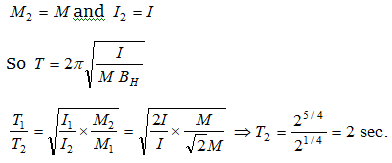# NEET Questions Solved

A vibration magnetometer consists of two identical bar magnets placed one over the other such that they are perpendicular and bisect each other. The time period of oscillation in a horizontal magnetic field is ${2}^{5}{4}}$ seconds. One of the magnets is removed and if the other magnet oscillates in the same field, then the time period in seconds is

(a) ${2}^{1}{4}}$                          (b) ${2}^{1}{2}}$

(c) 2                              (d) ${2}^{3}{4}}$

Initially magnetic moment of system ${M}_{1}=\sqrt{{M}^{2}+{M}^{2}}=\sqrt{2}M$

and the moment of inertia ${I}_{1}=I+I=2I$

Finally when one of the magnet is removed thenDifficulty Level:

• 17%
• 21%
• 21%
• 43%